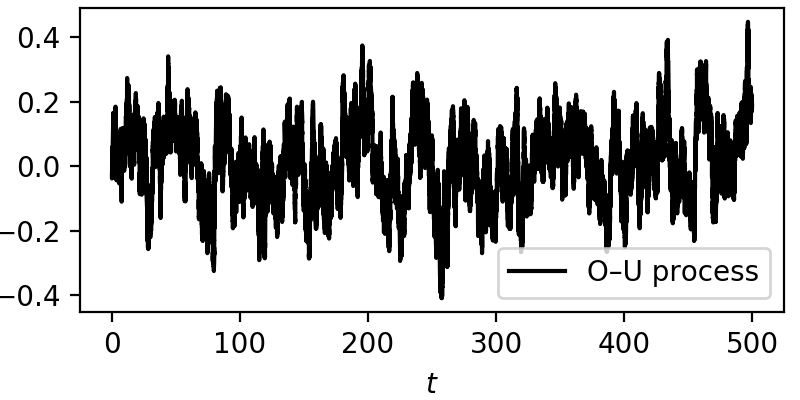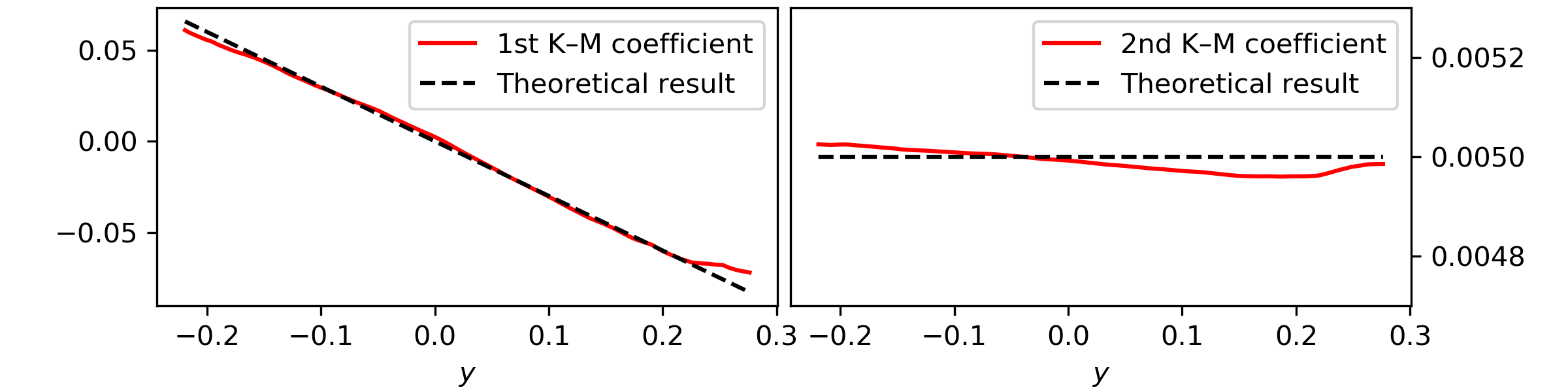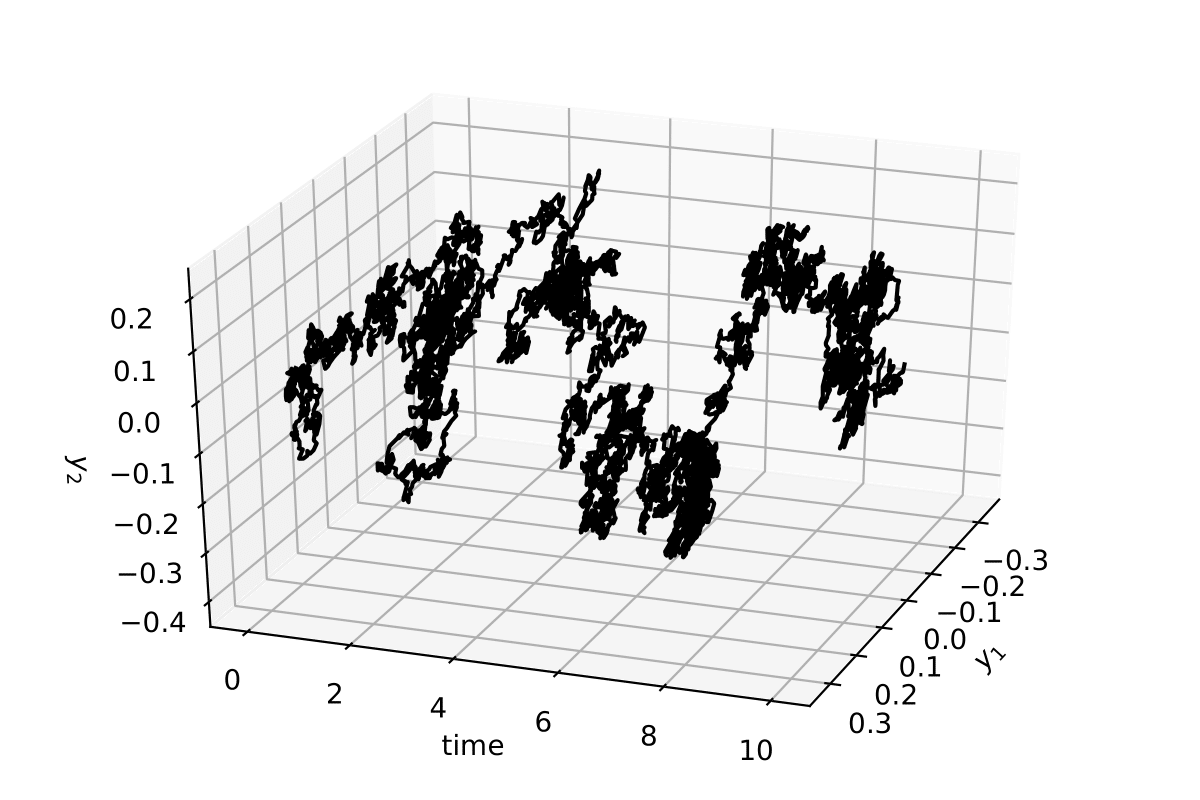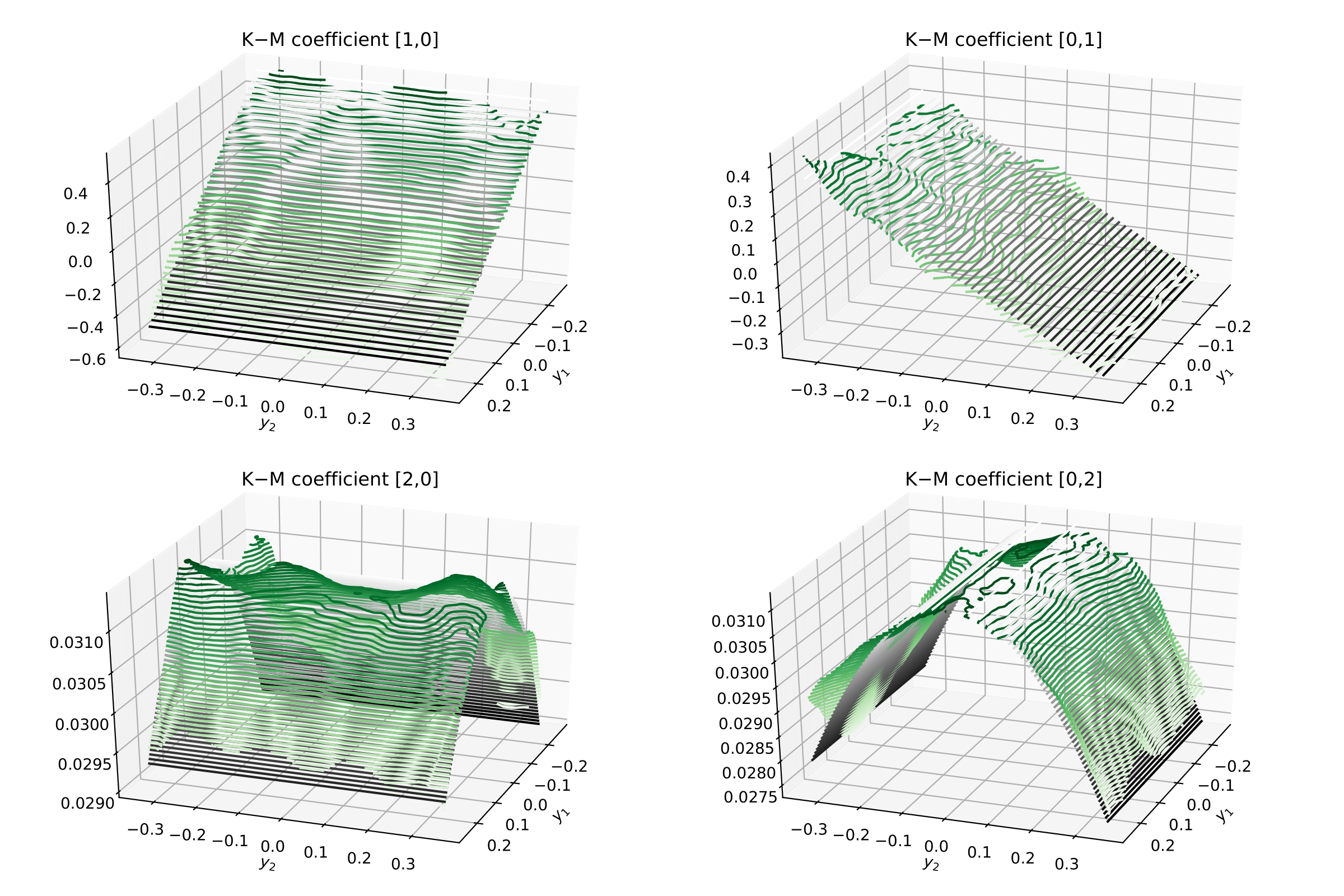# Kramers—Moyal¶

kramersmoyal is a python package designed to obtain the Kramers—Moyal coefficients, or conditional moments, from stochastic data of any dimension. It employs kernel density estimations, instead of a histogram approach, to ensure better results for low number of points as well as allowing better fitting of the results.

# Installation¶

To install kramersmoyal, just use pip

pip install kramersmoyal


Then on your favourite editor just use

from kramersmoyal import km


From here you can simply call

import numpy as np

# Number of bins
bins = np.array()

# Choose powers to calculate
powers = np.array([, ])

# And here x is your (1D, 2D, 3D) data
kmc, edge = km(x, bins = bins, powers = powers)


The library depends on numpy and scipy.

# A one-dimensional stochastic process¶

## The theory¶

Take, for example, the well-documented one-dimension Ornstein—Uhlenbeck process, also known as Vašíček process. This process is governed by two main parameters: the mean-reverting parameter $$\theta$$ and the diffusion or volatility coefficient $$\sigma$$

$\mathrm{d}y(t) = -\theta y(t)\mathrm{d}t + \sigma \mathrm{d}W(t)$

which can be solved in various ways. For our purposes, recall that the drift coefficient, i.e., the first-order Kramers—Moyal coefficient, is given by $$\mathcal{M}^{}(y) = -\theta y$$ and the second-order Kramers—Moyal coefficient is $$\mathcal{M}^{}(y) = \sigma^2 / 2$$, i.e., the diffusion.

For this example let’s take $$\theta=0.3$$ and $$\sigma=0.1$$, over a total time of 500 units, with a sampling of 1000 Hertz, and from the generated data series retrieve the two parameters, the drift $$-\theta y(t)$$ and diffusion $$\sigma$$.

## Integrating an Ornstein—Uhlenbeck process¶

Here is a short code on generating a Ornstein—Uhlenbeck stochastic trajectory with a simple Euler–Maruyama integration method

# integration time and time sampling
t_final = 500
delta_t = 0.001

# The parameters theta and sigma
theta = 0.3
sigma = 0.1

# The time array of the trajectory
time = np.arange(0, t_final, delta_t)

# Initialise the array y
y = np.zeros(time.size)

# Generate a Wiener process
dw = np.random.normal(loc = 0, scale = np.sqrt(delta_t), size = time.size)

# Integrate the process
for i in range(1,time.size):
y[i] = y[i-1] - theta*y[i-1]*delta_t + sigma*dw[i]


From here we have a plain example of an Ornstein—Uhlenbeck process, always drifting back to zero, due to the mean-reverting drift $$-\theta y(t)$$. The effect of the noise can be seen across the whole trajectory.## Using kramersmoyal¶

Take the timeseries $$y(t)$$ and let’s study the Kramers—Moyal coefficients. For this let’s look at the drift and diffusion coefficients of the process, i.e., the first and second Kramers—Moyal coefficients, with an epanechnikov kernel

# Choose number of points of you target space
bins = np.array()

# Choose powers to calculate
powers = np.array([, ])

bw = 0.15

# The kmc holds the results, where edges holds the binning space
kmc, edges = km(y, kernel = kernels.epanechnikov, bw = bw, bins = bins, powers = powers)


This results inNotice here that to obtain the Kramers—Moyal coefficients you need to divide kmc by the timestep delta_t. This normalisation stems from the Taylor-like approximation, i.e., the Kramers—Moyal expansion ($$\delta t \to 0$$).

# A two-dimensional diffusion process¶

## Theory¶

A two-dimensional diffusion process is a stochastic process that comprises two $$W(t)$$ and allows for a mixing of these noise terms across its two dimensions.

$\begin{split}\begin{pmatrix} \mathrm{d}y_1(t) \\ \mathrm{d}y_2(t) \end{pmatrix}= \begin{pmatrix} N_1(y) \\ N_2(y) \end{pmatrix} \mathrm{d} t + \begin{pmatrix} g_{1,1}(y) & g_{1,2}(y) \\ g_{2,1}(y) & g_{2,2}(y) \end{pmatrix} \begin{pmatrix} \mathrm{d}W_1 \\ \mathrm{d}W_2 \end{pmatrix}\end{split}$

with $$N$$ the drift vector and $$g$$ the diffusion matrix, which can be state dependent. We define, as the previous example, a process identical to the Ornstein—Uhlenbeck process, with

$\begin{split}N = \begin{pmatrix} - N_1 y_1 \\ - N_2 y_2 \end{pmatrix}\end{split}$

and we take $$N_1=2.0$$ and $$N_2=1.0$$. For this particular case a more involved diffusion matrix $$g$$ will be used. Let the matrix $$g$$ be state-dependent, i.e., dependent of the actual values of $$y_1$$ and $$y_2$$ via

$\begin{split}g = \begin{pmatrix} \frac{g_{1,1} }{1+e^{y_1^2}} & g_{1,2} \\ g_{2,1} & \frac{g_{2,2} }{1+e^{y_2^2}} \end{pmatrix}\end{split}$

and we will take $$g_{1,1} = g_{2,2}=0.5$$ and $$g_{1,2} = g_{2,1} = 0$$.

## Integrating a 2-dimensional process¶

Taking the above parameters and writing again an Euler–Maruyama integration method

# integration time and time sampling
t_final = 2000
delta_t = 0.001

# Define the drift vector N
N = np.array([2.0, 1.0])

# Define the diffusion matrix g
g = np.array([[0.5, 0.0], [0.0, 0.5]])

# The time array of the trajectory
time = np.arange(0, t_final, delta_t)

# Initialise the array y
y = np.zeros([time.size, 2])

# Generate two Wiener processes with a scale of np.sqrt(delta_t)
dW = np.random.normal(loc = 0, scale = np.sqrt(delta_t), size = [time.size, 2])

# Integrate the process (takes about 20 secs)
for i in range(1, time.size):
y[i,0] = y[i-1,0]  -  N * y[i-1,0] * delta_t + g[0,0]/(1 + np.exp(y[i-1,0]**2)) * dW[i,0]  +  g[0,1] * dW[i,1]
y[i,1] = y[i-1,1]  -  N * y[i-1,1] * delta_t + g[1,0] * dW[i,0]  +  g[1,1]/(1 + np.exp(y[i-1,1]**2)) * dW[i,1]


The stochastic trajectory in 2 dimensions for 10 time units (10000 data points)## Back to kramersmoyal and the Kramers—Moyal coefficients¶

First notice that all the results now will be two-dimensional surfaces, so we will need to plot them as such

# Choose the size of your target space in two dimensions
bins = np.array([300, 300])

# Introduce the desired orders to calculate, but in 2 dimensions
powers = np.array([[0,0], [1,0], [0,1], [1,1], [2,0], [0,2], [2,2]])
# insert into kmc:   0      1      2      3      4      5      6

# Notice that the first entry in [,] is for the first dimension, the
# second for the second dimension...

# Choose a desired bandwidth bw
bw = 0.1

# Calculate the Kramers−Moyal coefficients
kmc, edges = km(y, bw = bw, bins = bins, powers = powers)

# The K−M coefficients are stacked along the first dim of the
# kmc array, so kmc[1,...] is the first K−M coefficient, kmc[2,...]
# is the second. These will be 2-dimensional matrices.


Now one can visualise the Kramers–Moyal coefficients (surfaces) in green and the respective theoretical surfaces in black. (Don’t forget to normalise: kmc / delta_t).# Literature¶

1 Friedrich, R., Peinke, J., Sahimi, M., Tabar, M. R. R. Approaching complexity by stochastic methods: From biological systems to turbulence, [Phys. Rep. 506, 87–162 (2011)](https://doi.org/10.1016/j.physrep.2011.05.003).

The study of stochastic processes from a data-driven approach is grounded in extensive mathematical work. From the applied perspective there are several references to understand stochastic processes, the Fokker—Planck equations, and the Kramers—Moyal expansion

Tabar, M. R. R. (2019). Analysis and Data-Based Reconstruction of Complex Nonlinear Dynamical Systems. Springer, International Publishing
Risken, H. (1989). The Fokker–Planck equation. Springer, Berlin, Heidelberg.
Gardiner, C.W. (1985). Handbook of Stochastic Methods. Springer, Berlin.

An extensive review on the subject can be found here.

# Funding¶

Helmholtz Association Initiative Energy System 2050 - A Contribution of the Research Field Energy and the grant No. VH-NG-1025 and STORM - Stochastics for Time-Space Risk Models project of the Research Council of Norway (RCN) No. 274410.